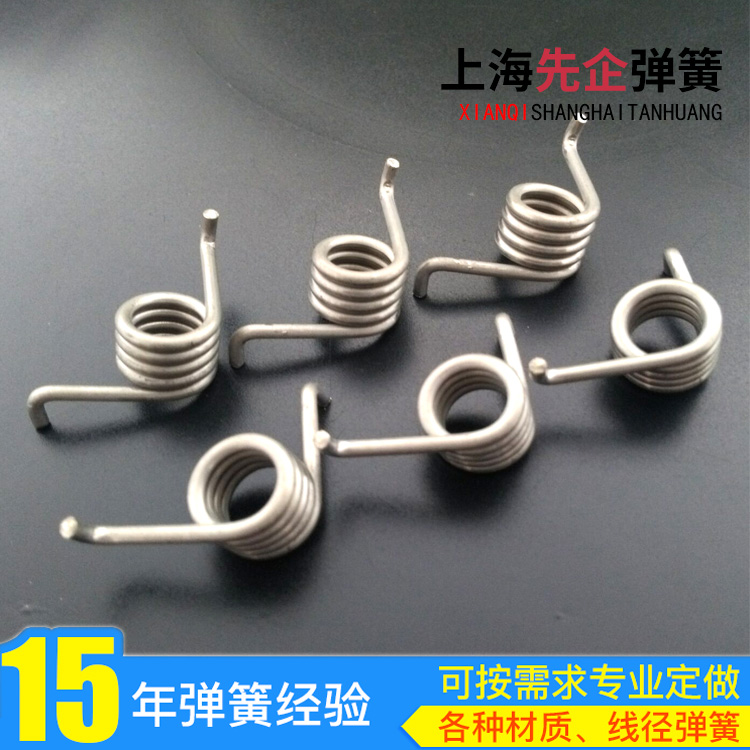# 扭力弹簧的扭力怎样计算E=线材之钢性模数：琴钢丝E=21000 ,不锈钢丝E=19400 ,磷青铜线E=11200 ,黄铜线E=11200

d=线径 Do=OD=外径 Di=ID=内径 Dm=MD=中径=Do-d N=总圈数 R=负荷作用的力臂 p=3.1416

【相关推荐】

• 服务热线

18116101059